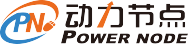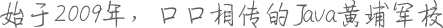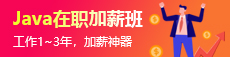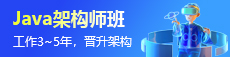# Java质数算法介绍

Java质数算法是什么？动力节点小编来为大家解答。质数就是一个数只可以被它自己和1整除(1不是质数)

## 1.判断一个数是不是质数

``````	public static int F(int x) {   //判断是否为质数 2，3，5，7，11，13，17，19......
if(x==1) return 0;
for(int i=2;i<=x/i;i++)
{
System.out.println(x+ " "+ i);
if(x%i==0)
return 0;
}
return 1;
}``````

## 2.分解质因数

``````	public static void prime(int n){
for(int i = 2; i <= n / i; i++){      循环到根号n为止
int a = 0, b = 0;
while(n % i == 0){                如果可以整除说明是质因数
a = i;
n /= i;                       一直除以这个数直到不整除为止
b++;                          累计a的个数
}
if(b > 0)
System.out.println(a + " " + b);
}
if(n > 1) System.out.println(n + " " + 1);
}``````
``````输入
24

2 3
3 1``````

## 3.埃氏筛选法

``````	static int st[] = new int ;        //0表示质数，1表示合数
static int n;
public static void E(int n) {
for(int i=2;i<=n;i++) {
if(st[i]==0)                    //第一轮，2开始，4，6，8，10，12...20被筛选
{                               //第二轮，3开始，6，9，12，15，18被筛选
for(int j=2*i;j<=n;j+=i)    //第三轮，5开始，10，15，20被筛选
st[j]=1;
}
}
}``````